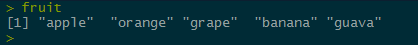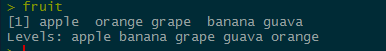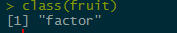# What is a factor? How would you create a factor in R?

Conceptually, factors are variables in R which take on a limited number of different values; such variables are often referred to as categorical variables. One of the most important use of factors is in statistical modeling; since categorical variables enter into statistical models differently than continuous variables, storing data as factors ensures that the modeling functions will treat such data correctly.

Initially, we have a character vector of names of fruits, let’s convert it into a factor:``````as.character(fruit)->fruit
``````let’s look at the class of the vector now: